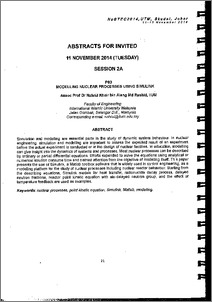# Modeling nuclear processes using simulink

Alang Md Rashid, Nahrul Khair (2014) Modeling nuclear processes using simulink. In: Nuclear Science Technology and Engineering Conference 2014 (NuSTEC14), 11-13 November 2014, Skudai, Johor. (Unpublished)Preview
PDF (Modeling nuclear processes using Simulink) - Accepted Version

## Abstract

Simulation and modeling are essential parts in the study of dynamic system behaviour. in nuclear engineering, simulation and modeling are important o assess the expected result of an experiment before the actual experiment is conducted or in the design of nuclear facilities. In education, modeling can give insight into the dynamics of systems and processes. Most nuclear processes can be described by ordinary or partial differential equations. Efforts expended to solve the equations using analytical or numerical solution consume time and distract attention from the objective of modeling itself. This paper presents the use of Simulink, a Matlab toolbox software that is widely used in control engineering, as a modeling platform for the study of nuclear processes including nuclear reactor behaviour. Starting from the describing equations, Simulink models for heat transfer, radionuclide decay process, delayed neutron fractions, reactor point kinetic equation with six delayed neutron group, and the effect of temperature feedback are used as examples.

Item Type: Conference or Workshop Item (UNSPECIFIED) 5928/40156 nuclear process, point kinetic equation, Simulink, matlab T Technology > TK Electrical engineering. Electronics Nuclear engineering Kulliyyah of Engineering > Department of Mechatronics Engineering Dr. Nahrul Khair Alang Md Rashid 30 Dec 2014 16:18 24 May 2018 14:09 http://irep.iium.edu.my/id/eprint/40156View Item